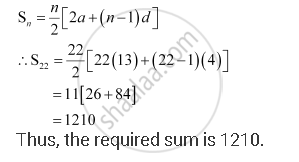CBSE (Arts) Class 11CBSE
Share
Notifications

View all notifications
Books Shortlist
Your shortlist is empty

# Find the Sum of All Two Digit Numbers Which When Divided by 4, Yields 1 as Remainder. - CBSE (Arts) Class 11 - Mathematics

Login
Create free account

Forgot password?
ConceptArithmetic Progression (A.P.)

#### Question

Find the sum of all two digit numbers which when divided by 4, yields 1 as remainder.

#### Solution

The two-digit numbers, which when divided by 4, yield 1 as remainder, are

13, 17, … 97.

This series forms an A.P. with first term 13 and common difference 4.

Let n be the number of terms of the A.P.

It is known that the nth term of an A.P. is given by, an = a + (n –1) d

∴97 = 13 + (n –1) (4)

⇒ 4 (n –1) = 84

⇒ n – 1 = 21

⇒ n = 22

Sum of n terms of an A.P. is given by,Is there an error in this question or solution?

#### APPEARS IN

NCERT Solution for Mathematics Textbook for Class 11 (2018 to Current)
Chapter 9: Sequences and Series
Q: 6 | Page no. 199
Solution Find the Sum of All Two Digit Numbers Which When Divided by 4, Yields 1 as Remainder. Concept: Arithmetic Progression (A.P.).
S